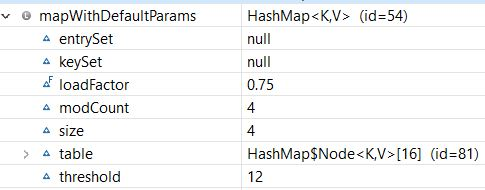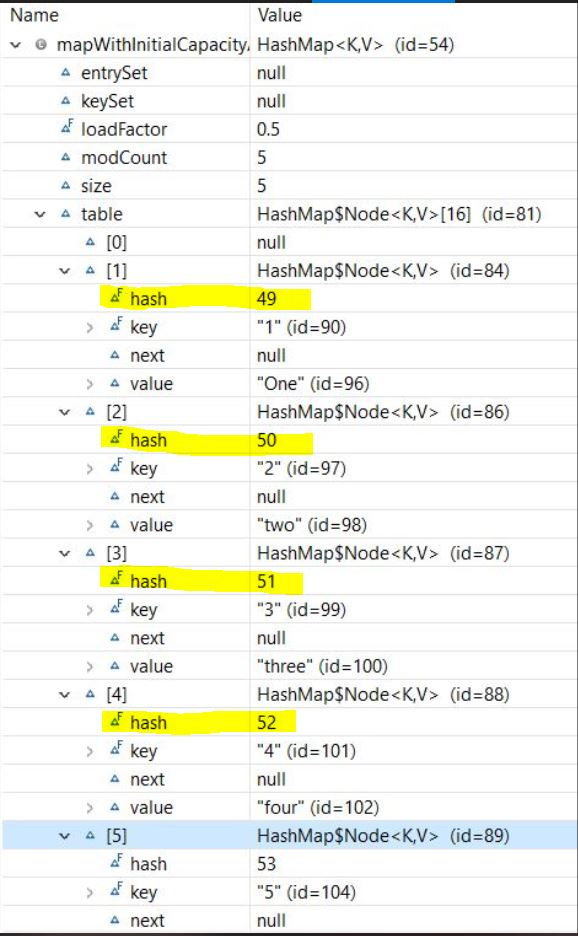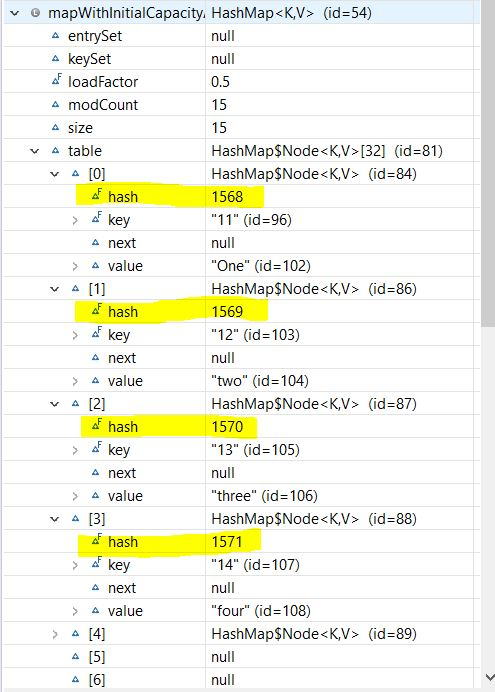### Get started with Spring 5 and Spring Boot 2, through the Learn Spring course:

>> CHECK OUT THE COURSE

## 1. Overview

In this article, we’ll see the significance of the load factor in Java’s HashMap and how it affects the map’s performance.

## 2. What Is HashMap?

The HashMap class belongs to the Java Collection framework and provides a basic implementation of the Map interface. We can use it when we want to store data in terms of key-value pairs. These key-value pairs are called map entries and are represented by the Map.Entry class.

## 3. HashMap Internals

Before discussing load factor, let’s review a few terms:

• hashing
• capacity
• threshold
• rehashing
• collision

HashMap works on the principle of hashing — an algorithm to map object data to some representative integer value. The hashing function is applied to the key object to calculate the index of the bucket in order to store and retrieve any key-value pair.

Capacity is the number of buckets in the HashMap. The initial capacity is the capacity at the time the Map is created. Finally, the default initial capacity of the HashMap is 16.

As the number of elements in the HashMap increases, the capacity is expanded. The load factor is the measure that decides when to increase the capacity of the Map. The default load factor is 75% of the capacity.

The threshold of a HashMap is approximately the product of current capacity and load factor. Rehashing is the process of re-calculating the hash code of already stored entries. Simply put, when the number of entries in the hash table exceeds the threshold, the Map is rehashed so that it has approximately twice the number of buckets as before.

A collision occurs when a hash function returns the same bucket location for two different keys.

Let’s create our HashMap:

``````Map<String, String> mapWithDefaultParams = new HashMap<>();
mapWithDefaultParams.put("1", "one");
mapWithDefaultParams.put("2", "two");
mapWithDefaultParams.put("3", "three");
mapWithDefaultParams.put("4", "four");
``````

Here is the structure of our Map:As we see, our HashMap was created with the default initial capacity (16) and the default load factor (0.75). Also, the threshold is 16 * 0.75 = 12, which means that it will increase the capacity from 16 to 32 after the 12th entry (key-value-pair) is added.

## 4. Custom Initial Capacity and Load Factor

In the previous section, we created our HashMap with a default constructor. In the following sections, we’ll see how to create a HashMap passing the initial capacity and load factor to the constructor.

### 4.1. With Initial Capacity

First, let’s create a Map with the initial capacity:

``````Map<String, String> mapWithInitialCapacity = new HashMap<>(5);
``````

It will create an empty Map with the initial capacity (5) and the default load factor (0.75).

### 4.2. With Initial Capacity and Load Factor

Similarly, we can create our Map using both initial capacity and load factor:

``````Map<String, String> mapWithInitialCapacityAndLF = new HashMap<>(5, 0.5f);
``````

Here, it will create an empty Map with an initial capacity of 5 and a load factor of 0.5.

## 5. Performance

Although we have the flexibility to choose the initial capacity and the load factor, we need to pick them wisely. Both of them affect the performance of the Map. Let’s dig in into how these parameters are related to performance.

### 5.1. Complexity

As we know, HashMap internally uses hash code as a base for storing key-value pairs. If the hashCode() method is well-written, HashMap will distribute the items across all the buckets. Therefore, HashMap stores and retrieves entries in constant time O(1).

However, the problem arises when the number of items is increased and the bucket size is fixed. It will have more items in each bucket and will disturb time complexity.

The solution is that we can increase the number of buckets when the number of items is increased. We can then redistribute the items across all the buckets. In this way, we’ll be able to keep a constant number of items in each bucket and maintain the time complexity of O(1).

Here, the load factor helps us to decide when to increase the number of buckets. With a lower load factor, there will be more free buckets and, hence, fewer chances of a collision. This will help us to achieve better performance for our Map. Hence, we need to keep the load factor low to achieve low time complexity.

A HashMap typically has a space complexity of O(n), where n is the number of entries. A higher value of load factor decreases the space overhead but increases the lookup cost.

### 5.2. Rehashing

When the number of items in the Map crosses the threshold limit, the capacity of the Map is doubled. As discussed earlier, when capacity is increased, we need to equally distribute all the entries (including existing entries and new entries) across all buckets. Here, we need rehashing. That is, for each existing key-value pair, calculate the hash code again with increased capacity as a parameter.

Basically, when the load factor increases, the complexity increases. Rehashing is done to maintain a low load factor and low complexity for all the operations.

Let’s initialize our Map:

``````Map<String, String> mapWithInitialCapacityAndLF = new HashMap<>(5,0.75f);
mapWithInitialCapacityAndLF.put("1", "one");
mapWithInitialCapacityAndLF.put("2", "two");
mapWithInitialCapacityAndLF.put("3", "three");
mapWithInitialCapacityAndLF.put("4", "four");
mapWithInitialCapacityAndLF.put("5", "five");``````

And let’s take a look at the structure of the Map:Now, let’s add more entries to our Map:

``````mapWithInitialCapacityAndLF.put("6", "Six");
mapWithInitialCapacityAndLF.put("7", "Seven");
//.. more entries
mapWithInitialCapacityAndLF.put("15", "fifteen");``````

And let’s observe our Map structure again:Although rehashing helps to keep low complexity, it’s an expensive process. If we need to store a huge amount of data, we should create our HashMap with sufficient capacity. This is more efficient than automatic rehashing.

### 5.3. Collision

Collisions may occur due to a bad hash code algorithm and often slow down the performance of the Map.

Prior to Java 8, HashMap in Java handles collision by using LinkedList to store map entries. If a key ends up in the same bucket where another entry already exists, it’s added at the head of the LinkedList. In the worst case, this will increase complexity to O(n).

To avoid this issue, Java 8 and later versions use a balanced tree (also called a red-black tree) instead of a LinkedList to store collided entries. This improves the worst-case performance of HashMap from O(n) to O(log n).

HashMap initially uses the LinkedList. Then when the number of entries crosses a certain threshold, it will replace a LinkedList with a balanced binary tree. The TREEIFY_THRESHOLD constant decides this threshold value. Currently, this value is 8, which means if there are more than 8 elements in the same bucket, Map will use a tree to hold them.

## 6. Conclusion

In this article, we discussed one of the most popular data structures: HashMap. We also saw how the load factor together with capacity affects its performance.

As always, the code examples for this article are available over on GitHub.

### Get started with Spring 5 and Spring Boot 2, through the Learn Spring course:

>> CHECK OUT THE COURSE